Skip to content →

# Tag: hyperbolic

Here I will go over the last post at a more leisurely pace, focussing on a couple of far more trivial examples. Here’s the goal : we want to assign a quiver-superpotential to any subgroup of finite index of the modular group. So fix such a subgroup $\Gamma’$ of the modular group $\Gamma=PSL_2(\mathbb{Z})$ and consider the associated permutation representation of $\Gamma$ on the left-cosets $\Gamma/\Gamma’$. As $\Gamma \simeq C_2 \ast C_3$ this representation is determined by the action of the order 2 and order 3 generators of the modular group. There are a number of combinatorial gadgets to control the subgroup $\Gamma’$ and the associated permutation representation : (generalized) Farey symbols and dessins d’enfants.

Recall that the modular group acts on the upper-halfplane (the ‘hyperbolic plane’) by Moebius transformations, so to any subgroup $\Gamma’$ we can associate a fundamental domain for its restricted action. The dessins and the Farey symbols give us a particular choice of these fundamental domains. Let us consider the two most trivial subgroups of all : the modular group itself (so $\Gamma/\Gamma$ is just one element and therefore the associated permutation representation is just the trivial representation) and the unique index two subgroup $\Gamma_2$ (so there are two cosets $\Gamma/\Gamma_2$ and the order 2 generator interchanges these two while the order 3 generator acts trivially on them). The fundamental domains of $\Gamma$ (left) and $\Gamma_2$ (right) are depicted below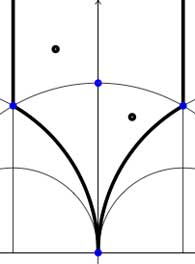In both cases the fundamental domain is bounded by the thick black (hyperbolic) edges. The left-domain consists of two hyperbolic triangles (the upper domain has $\infty$ as the third vertex) and the right-domain has 4 triangles. In general, if the subgroup $\Gamma’$ has index n, then its fundamental domain will consist of $2n$ hyperbolic triangles. Note that these triangles are part of the Dedekind tessellation so really depict the action of $PGL_2(\mathbb{Z}$ and any $\Gamma$-hyperbolic triangle consists of one black and one white triangle in Dedekind’s coloring. We will indicate the color of a triangle by a black circle if the corresponding triangle is black. Of course, the bounding edges of the fundamental domain need to be identified and the Farey symbol is a notation device to clarify this. The Farey symbols of the above domains are
$$\xymatrix{\infty \ar@{-}[r]_{\circ} & 0 \ar@{-}[r]_{\bullet} & \infty}$$ and $$\xymatrix{\infty \ar@{-}[r]_{\bullet} & 0 \ar@{-}[r]_{\bullet} & \infty}$$ respectively. In both cases this indicates that the two bounding edges on the left are to be identified as are the two bounding edges on the right (so, in particular, after identification $\infty$ coincides with $0$). Hence, after identification, the $\Gamma$ domain consists of two triangles on the vertices ${ 0,i,\rho }$ (where $\rho=e^{2 \pi i}{6}$) (the blue dots) sharing all three edges, the $\Gamma_2$ domain consists of 4 triangles on the 4 vertices ${ 0,i,\rho,\rho^2 }$ (the blue dots). In general we have three types of vertices : cusps (such as 0 or $\infty$), even vertices (such as $i$ where there are 4 hyperbolic edges in the Dedekind tessellation) and odd vertices (such as $\rho$ and $\rho^2$ where there are 6 hyperbolic edges in the tessellation).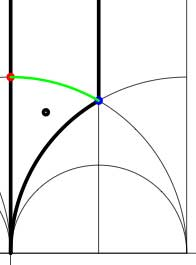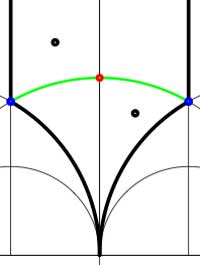Another combinatorial gadget assigned to the fundamental domain is the cuboid tree diagram or dessin. It consists of all odd and even vertices on the boundary of the domain, together with all odd and even vertices in the interior. These vertices are then connected with the hyperbolic edges connecting them. If we color the even vertices red and the odds blue we have the indicated dessins for our two examples (the green pictures). An half-edge is an edge connecting a red and a blue vertex in the dessin and we number all half-edges. So, the $\Gamma$-dessin has 1 half-edge whereas the $\Gamma_2$-dessin has two (in general, the number of these half-edges is equal to the index of the subgroup). Observe also that every triangle has exactly one half-edge as one of its three edges. The dessin gives all information to calculate the permutation representation on the coset-set $\Gamma/\Gamma’$ : the action of the order 2 generator of $\Gamma$ is given by taking for each internal red vertex the two-cycle $~(a,b)$ where a and b are the numbers of the two half-edges connected to the red vertex and the action of the order 3 generator is given by taking for every internal blue vertex the three cycle $~(c,d,e)$ where c, d and e are the numbers of the three half-edges connected to the blue vertex in counter-clockwise ordering. Our two examples above are a bit too simplistic to view this in action. There are no internal blue vertices, so the action of the order 3 generator is trivial in both cases. For $\Gamma$ there is also no red internal vertex, whence this is indeed the trivial representation whereas for $\Gamma_2$ there is one internal red vertex, so the action of the order 2 generator is given by $~(1,2)$, which is indeed the representation representation on $\Gamma/\Gamma_2$. In general, if the index of the subgroup $\Gamma’$ is n, then we call the subgroup of the symmetric group on n letters $S_n$ generated by the action-elements of the order 2 and order 3 generator the monodromy group of the permutation representation (or of the subgroup). In the trivial cases here, the monodromy groups are the trivial group (for $\Gamma$) and $C_2$ (for $\Gamma_2$).

As a safety-check let us work out all these concepts in the next simplest examples, those of some subgroups of index 3. Consider the Farey symbols

$$\xymatrix{\infty \ar@{-}[r]_{\circ} & 0 \ar@{-}[r]_{\circ} & 1 \ar@{-}[r]_{\circ} & \infty}$$ and
$$\xymatrix{\infty \ar@{-}[r]_{\circ} & 0 \ar@{-}[r]_{1} & 1 \ar@{-}[r]_{1} & \infty}$$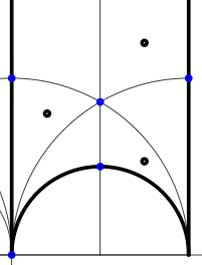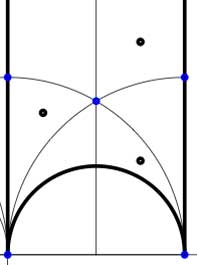In these cases the fundamental domain consists of 6 triangles with the indicated vertices (the blue dots). The distinction between the two is that in the first case, one identifies the two edges of the left, resp. bottom, resp. right boundary (so, in particular, 0,1 and $\infty$ are identified) whereas in the second one identifies the two edges of the left boundary and identifies the edges of the bottom with those of the right boundary (here, 0 is identified only with $\infty$ but also $1+i$ is indetified with $\frac{1}{2}+\frac{1}{2}i$).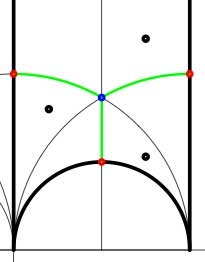In both cases the dessin seems to be the same (and given by the picture on the right). However, in the first case all three red vertices are distinct hence there are no internal red vertices in this case whereas in the second case we should identify the bottom and right-hand red vertex which then becomes an internal red vertex of the dessin!

Hence, if we order the three green half-edges 1,2,3 starting with the bottom one and counting counter-clockwise we see that in both cases the action of the order 3-generator of $\Gamma$ is given by the 3-cycle $~(1,2,3)$. The action of the order 2-generator is trivial in the first case, while given by the 2-cycle $~(1,2)$ in the second case. Therefore, the monodromy group is the cylic group $C_3$ in the first case and is the symmetric group $S_3$ in the second case.

Next time we will associate a quiver to these vertices and triangles as well as a cubic superpotential which will then allow us to define a noncommutative algebra associated to any subgroup of the modular group. The monodromy group of the situation will then reappear as a group of algebra-automorphisms of this noncommutative algebra!

Yesterday, Jan Stienstra gave a talk at theARTS entitled “Quivers, superpotentials and Dimer Models”. He started off by telling that the talk was based on a paper he put on the arXiv Hypergeometric Systems in two Variables, Quivers, Dimers and Dessins d’Enfants but that he was not going to say a thing about dessins but would rather focuss on the connection with superpotentials instead…pleasing some members of the public, while driving others to utter despair.

Anyway, it gave me the opportunity to figure out for myself what dessins might have to do with dimers, whathever these beasts are. Soon enough he put on a slide containing the definition of a dimer and from that moment on I was lost in my own thoughts… realizing that a dessin d’enfant had to be a dimer for the Dedekind tessellation of its associated Riemann surface!
and a few minutes later I could slap myself on the head for not having thought of this before :

There is a natural way to associate to a Farey symbol (aka a permutation representation of the modular group) a quiver and a superpotential (aka a necklace) defining (conjecturally) a Calabi-Yau algebra! Moreover, different embeddings of the cuboid tree diagrams in the hyperbolic plane may (again conjecturally) give rise to all sorts of arty-farty fanshi-wanshi dualities…

I’ll give here the details of the simplest example I worked out during the talk and will come back to general procedure later, when I’ve done a reference check. I don’t claim any originality here and probably all of this is contained in Stienstra’s paper or in some physics-paper, so if you know of a reference, please leave a comment. Okay, remember the Dedekind tessellation ?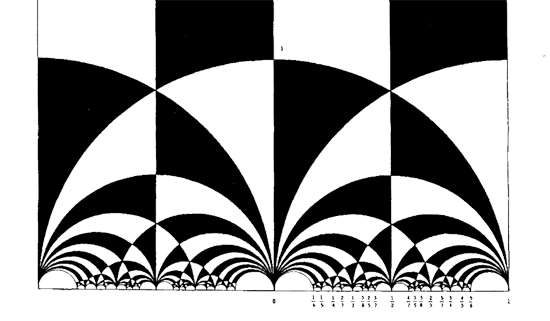So, all hyperbolic triangles we will encounter below are colored black or white. Now, take a Farey symbol and consider its associated special polygon in the hyperbolic plane. If we start with the Farey symbol

$$\xymatrix{\infty \ar@{-}_{(1)}[r] & 0 \ar@{-}_{\bullet}[r] & 1 \ar@{-}_{(1)}[r] & \infty}$$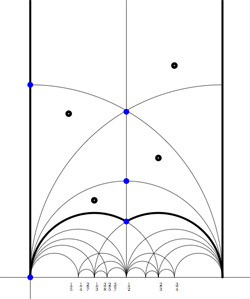we get the special polygonal region bounded by the thick edges, the vertical edges are identified as are the two bottom edges. Hence, this fundamental domain has 6 vertices (the 5 blue dots and the point at $i \infty$) and 8 hyperbolic triangles (4 colored black, indicated by a black dot, and 4 white ones).

Right, now let us associate a quiver to this triangulation (which embeds the quiver in the corresponding Riemann surface). The vertices of the triangulation are also the vertices of the quiver (so in our case we are going for a quiver with 6 vertices). Every hyperbolic edge in the triangulation gives one arrow in the quiver between the corresponding vertices. The orientation of the arrow is determined by the color of a triangle of which it is an edge : if the triangle is black, we run around its edges counter-clockwise and if the triangle is white we run over its edges clockwise (that is, the orientation of the arrow is independent of the choice of triangles to determine it). In our example, there is one arrows directed from the vertex at $i$ to the vertex at $0$, whether you use the black triangle on the left to determine the orientation or the white triangle on the right. If we do this for all edges in the triangulation we arrive at the quiver below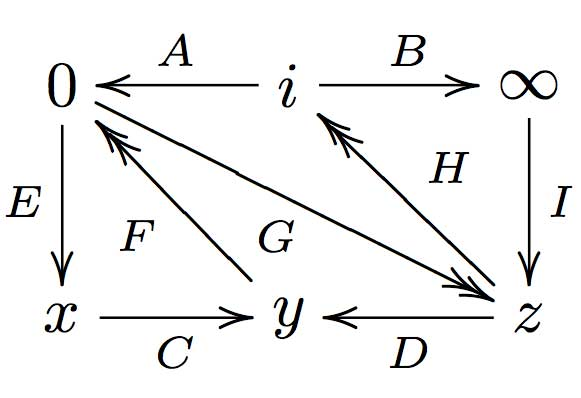where x,y and z are the three finite vertices on the $\frac{1}{2}$-axis from bottom to top and where I’ve used the physics-convention for double arrows, that is there are two F-arrows, two G-arrows and two H-arrows. Observe that the quiver is of Calabi-Yau type meaning that there are as much arrows coming into a vertex as there are arrows leaving the vertex.

Now that we have our quiver we determine the superpotential as follows. Fix an orientation on the Riemann surface (for example counter-clockwise) and sum over all black triangles the product of the edge-arrows counterclockwise MINUS sum over all white triangles
the product of the edge arrows counterclockwise. So, in our example we have the cubic superpotential

$IH’B+HAG+G’DF+FEC-BHI-H’G’A-GFD-CEF’$

From this we get the associated noncommutative algebra, which is the quotient of the path algebra of the above quiver modulo the following ‘commutativity relations’

$\begin{cases} GH &=G’H’ \\ IH’ &= IH \\ FE &= F’E \\ F’G’ &= FG \\ CF &= CF’ \\ EC &= GD \\ G’D &= EC \\ HA &= DF \\ DF’ &= H’A \\ AG &= BI \\ BI &= AG’ \end{cases}$

and morally this should be a Calabi-Yau algebra (( can someone who knows more about CYs verify this? )). This concludes the walk through of the procedure. Summarizing : to every Farey-symbol one associates a Calabi-Yau quiver and superpotential, possibly giving a Calabi-Yau algebra!

Here the details of the iguanodon series. Start with the Farey sequence $F(n)$of order n which is the sequence of completely reduced fractions between 0 and 1 which, when in lowest terms, have denominators less than or equal to n, arranged in order of increasing size. Here are the first eight Fareys

F(1) = {0⁄1, 1⁄1}
F(2) = {0⁄1, 1⁄2, 1⁄1}
F(3) = {0⁄1, 1⁄3, 1⁄2, 2⁄3, 1⁄1}
F(4) = {0⁄1, 1⁄4, 1⁄3, 1⁄2, 2⁄3, 3⁄4, 1⁄1}
F(5) = {0⁄1, 1⁄5, 1⁄4, 1⁄3, 2⁄5, 1⁄2, 3⁄5, 2⁄3, 3⁄4, 4⁄5, 1⁄1}
F(6) = {0⁄1, 1⁄6, 1⁄5, 1⁄4, 1⁄3, 2⁄5, 1⁄2, 3⁄5, 2⁄3, 3⁄4, 4⁄5, 5⁄6, 1⁄1}
F(7) = {0⁄1, 1⁄7, 1⁄6, 1⁄5, 1⁄4, 2⁄7, 1⁄3, 2⁄5, 3⁄7, 1⁄2, 4⁄7, 3⁄5, 2⁄3, 5⁄7, 3⁄4, 4⁄5, 5⁄6, 6⁄7, 1⁄1}
F(8) = {0⁄1, 1⁄8, 1⁄7, 1⁄6, 1⁄5, 1⁄4, 2⁄7, 1⁄3, 3⁄8, 2⁄5, 3⁄7, 1⁄2, 4⁄7, 3⁄5, 5⁄8, 2⁄3, 5⁄7, 3⁄4, 4⁄5, 5⁄6, 6⁄7, 7⁄8, 1⁄1}Farey sequences have plenty of mysterious properties. For example, in 1924 J. Franel and Edmund Landau proved that an asymptotic density result about Farey sequences is equivalent to the Riemann hypothesis.
More precisely, let a(n) be the number of terms in the Farey sequence F(n) (that is, a(1)=2,a(2)=3,…,a(8)=23 etc. This is sequence A005728 in the online integer sequences catalog).
Let $F(n)_j$ denote the j-th term in F(n), then the following conjecture is equivalent to the Riemann hypothesis

For every $\epsilon > 0$ there is a constant C depending on $\epsilon$ such that

$\sum_{j=1}^{a(n)} | F(n)_j – \frac{j}{a(n)} | < C n^{\frac{1}{2}+\epsilon}$

when n goes to infinity. Anyway, let us continue our construction. Farey sequences are clearly symmetric around 1/2 so let us just take half of them, so we jump to 1 when we have reached 1/2. Let us extend this halved Farey on both sides with $\infty$ and call it the modified Farey sequence f(n). For example,

$f(3) = {~\infty,0,\frac{1}{3},\frac{1}{2},1,\infty }$

Now consider the Farey code in which we identify the two sides connected to $\infty$ and mark two consecutive Farey numbers as

$$\xymatrix{f(n)_i \ar@{-}[r]_{\bullet} & f(n)_{i+1}}$$

That is, the Farey code associated to the modified sequence f(3) is

$$\xymatrix{\infty \ar@{-}[r]_{1} & 0 \ar@{-}[r]_{\bullet} & \frac{1}{3} \ar@{-}[r]_{\bullet} & \frac{1}{2} \ar@{-}[r]_{\bullet} & 1 \ar@{-}[r]_{1} & \infty}$$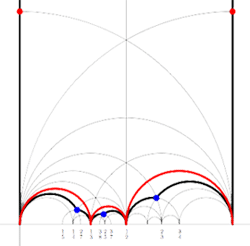Recall from earlier that to a Farey-code we can associate a special polygon by first taking the hyperbolic convex hull of all the terms in the sequence (the region bounded by the vertical lines and the bottom red circles in the picture on the left) and adding to it for each odd interval $$\xymatrix{f(n)_i \ar@{-}[r]_{\bullet} & f(n)_{i+1}}$$ the triangle just outside the convex hull consisting of two odd edges in the Dedekind tessellation (then we obtain the region bounded by the black geodesics for the sequence f(3)).

Next, we can associate to this special polygon a cuboid tree diagram by considering all even and odd vertices on the boundary (which are tinted red, respectively blue) together with all odd vertices in the interior of the special polygon. These are indicated in the left picture below. If we connect these vertices with the geodesics in the polygon we get a cuboid tree diagram. The obtained cuboid tree diagram is depicted on the right below.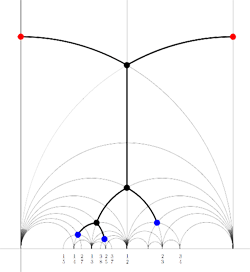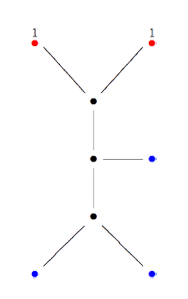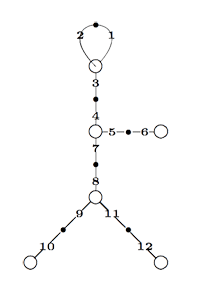Finally, identifying the red points (as they lie on geodesics connected to $\infty$ which are identified in the Farey code), adding even points on the remaining geodesics and numbering the obtained half-lines we obtain the dessin d’enfant given on the left hand side. To such a dessin we can associate its monodromy group which is a permutation group on the half-lines generated by an order two element indicating which half-lines make up a line and an order three element indicating which half-lines one encounters by walking counter-clockwise around a three-valent vertex. For the dessin on the left the group is therefore the subgroup of $S_{12}$ generated by the elements

$\alpha = (1,2)(3,4)(5,6)(7,8)(9,10)(11,12)$

$\beta = (1,2,3)(4,5,7)(8,9,11)$

and a verification with GAP tells us that this group is the sporadic Mathieu group $M_{12}$. This concludes the description of the second member of the Iguanodon series. If you like to check that the first 8 iguanodons are indeed the simple groups

$L_2(7), M_{12}, A_{16}, M_{24}, A_{28}, A_{40}, A_{48}, A_{60}, \ldots$

the following dissection of the Iguanodon may prove useful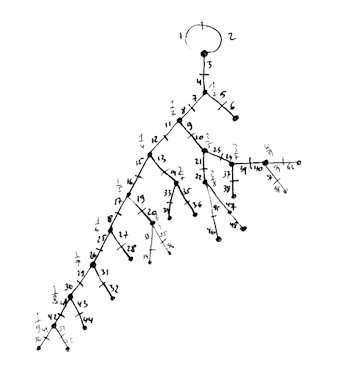If you only tune in now, you might want to have a look at the definition of Mathieu’s blackjack and the first part of the proof of the Conway-Ryba winning strategy involving the Steiner system S(5,6,12) and the Mathieu sporadic group $M_{12}$.

We’re trying to disprove the existence of misfits, that is, of non-hexad positions having a total value of at least 21 such that every move to a hexad would increase the total value. So far, we succeeded in showing that such a misfit must have the patern

$\begin{array}{|c|ccc|} \hline 6 & III & \ast & 9 \\ 5 & II & 7 & . \\ IV & I & 8 & . \\ \hline & & & \end{array}$

That is, a misfit must contain the 0-card (queen) and cannot contain the 10 or 11(jack) and must contain 3 of the four Romans. Now we will see that a misfit also contains precisely one of {5,6} (and consequently also exactly one card from {7,8,9}). To start, it is clear that it cannot contain BOTH 5 and 6 (then its total value can be at most 20). So we have to disprove that a misfit can miss {5,6} entirely (and so the two remaining cards (apart from the zero and the three Romans) must all belong to {7,8,9}).

Lets assume the misfit misses 5 and 6 and does not contain 9. Then, it must contain 4 (otherwise, its column-distribution would be (0,3,3,0) and it would be a hexad). There are just three such positions possible

$\begin{array}{|c|ccc|} \hline . & \ast & \ast & . \\ . & \ast & \ast & . \\ \ast & . & \ast & . \\ \hline – & – & ? & ? \end{array}$ $\begin{array}{|c|ccc|} \hline . & \ast & \ast & . \\ . & . & \ast & . \\ \ast & \ast & \ast & . \\ \hline – & + & ? & ? \end{array}$ $\begin{array}{|c|ccc|} \hline . & . & \ast & . \\ . & \ast & \ast & . \\ \ast & \ast & \ast & . \\ \hline – & 0 & ? & ? \end{array}$

Neither of these can be misfits though. In the first one, there is an 8->5 move to a hexad of smaller total value (in the second a 7->5 move and in the third a 7->6 move). Right, so the 9 card must belong to a misfit. Assume it does not contain the 4-card, then part of the misfit looks like (with either a 7- or an 8-card added)

$\begin{array}{|c|ccc|} \hline . & \ast & \ast & \ast \\ . & \ast & ? & . \\ . & \ast & ? & . \\ \hline & & & \end{array}$ contained in the unique hexad $\begin{array}{|c|ccc|} \hline \ast & \ast & \ast & \ast \\ . & \ast & & . \\ . & \ast & & . \\ \hline & & & \end{array}$

Either way the moves 7->6 or 8->6 decrease the total value, so it cannot be a misfit. Therefore, a misfit must contain both the 4- and 9-card. So it is of the form on the left below

$\begin{array}{|c|ccc|} \hline . & ? & \ast & \ast \\ . & ? & ? & . \\ \ast & ? & ? & . \\ \hline & & & \end{array}$ $\begin{array}{|c|ccc|} \hline . & . & \ast & . \\ . & \ast & \ast & \ast \\ \ast & \ast & . & . \\ \hline – & 0 & – & + \end{array}$ $\begin{array}{|c|ccc|} \hline . & . & \ast & \ast \\ . & \ast & \ast & . \\ \ast & \ast & . & . \\ \hline & & & \end{array}$

If this is a genuine misfit only the move 9->10 to a hexad is possible (the move 9->11 is not possible as all BUT ONE of {0,1,2,3,4} is contained in the misfit). Now, the only hexad containing 0,4,10 and 2 from {1,2,3} is in the middle, giving us what the misfit must look like before the move, on the right. Finally, this cannot be a misfit as the move 7->5 decreases the total value.

That is, we have proved the claim that a misfit must contain one of {5,6} and one of {7,8,9}. Right, now we can deliver the elegant finishing line of the Kahane-Ryba proof. A misfit must contain 0 and three among {1,2,3,4} (let us call the missing card s), one of $5+\epsilon$ with $0 \leq \epsilon \leq 1$ and one of $7+\delta$ with $0 \leq \delta \leq 2$. Then, the total value of the misfit is

$~(0+1+2+3+4-s)+(5+\epsilon)+(7+\delta)=21+(1+\delta+\epsilon-s)$

So, if this value is strictly greater than 21 (and we will see in a moment is has to be if it is at least 21) then we deduce that $s < 1 + \delta + \epsilon \leq 4$. Therefore $1+\delta+\epsilon$ belongs to the misfit. But then the move $1+\delta \epsilon \rightarrow s$ moves the misfit to a 6-tuple with total value 21 and hence (as we see in a moment) must be a hexad and hence this is a decreasing move! So, finally, there are no misfits!

Hence, from every non-hexad pile of total value at least 21 we have a legal move to a hexad. Because the other player cannot move from an hexad to another hexad we are done with our strategy provided we can show (a) that the total value of any hexad is at least 21 and (b) that ALL 6-piles of total value 21 are hexads. As there are only 132 hexads it is easy enough to have their sum-distribution. Here it is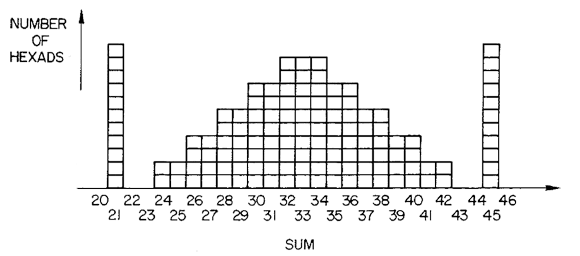That is, (a) is proved by inspection and we see that there are 11 hexads of sum 21 (the light hexads in Conway-speak) and there are only 11 ways to get 21 as a sum of 6 distinct numbers from {0,1,..,11} so (b) follows. Btw. the obvious symmetry of the sum-distribution is another consequence of the duality t->11-t discussed briefly at the end of part 2.

Clearly, I’d rather have conceptual proofs for all these facts and briefly tried my hand. Luckily I did spot the following phrase on page 326 of Conway-Sloane (discussing the above distribution) :

“It will not be easy to explain all the above observations. They are certainly connected with hyperbolic geometry and with the ‘hole’ structure of the Leech lattice.”

So, I’d better leave it at this…

References

Joseph Kahane and Alexander J. Ryba, “The hexad game

John H. Conway and N. J.A. Sloane, “Sphere packings, Lattices and Groups” chp. 11 ‘The Golay codes and the Mathieu groups’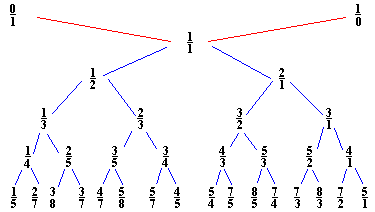John Farey (1766-1826) was a geologist of sorts. Eyles, quoted on the math-biographies site described his geological work as

“As a geologist Farey is entitled to respect for the work which he carried out himself, although it has scarcely been noticed in the standard histories of geology.”

That we still remember his name after 200 years is due to a short letter he wrote in 1816 to the editor of the Philosophical Magazine

“On a curious Property of vulgar Fractions.
By Mr. J. Farey, Sen. To Mr. Tilloch

Sir. – On examining lately, some very curious and elaborate Tables of “Complete decimal Quotients,” calculated by Henry Goodwyn, Esq. of Blackheath, of which he has printed a copious specimen, for private circulation among curious and practical calculators, preparatory to the printing of the whole of these useful Tables, if sufficient encouragement, either public or individual, should appear to warrant such a step: I was fortunate while so doing, to deduce from them the following general property; viz.

If all the possible vulgar fractions of different values, whose greatest denominator (when in their lowest terms) does not exceed any given number, be arranged in the order of their values, or quotients; then if both the numerator and the denominator of any fraction therein, be added to the numerator and the denominator, respectively, of the fraction next but one to it (on either side), the sums will give the fraction next to it; although, perhaps, not in its lowest terms.

For example, if 5 be the greatest denominator given; then are all the possible fractions, when arranged, 1/5, 1/4, 1/3, 2/5, 1/2, 3/5, 2/3, 3/4, and 4/5; taking 1/3, as the given fraction, we have (1+1)/(5+3) = 2/8 = 1/4 the next smaller fraction than 1/3; or (1+1)/(3+2) = 2/5, the next larger fraction to 1/3. Again, if 99 be the largest denominator, then, in a part of the arranged Table, we should have 15/52, 28/97, 13/45, 24/83, 11/38, &c.; and if the third of these fractions be given, we have (15+13)/(52+45) = 28/97 the second: or (13+11)/(45+38) = 24/83 the fourth of them: and so in all the other cases.

I am not acquainted, whether this curious property of vulgar fractions has been before pointed out?; or whether it may admit of any easy or general demonstration?; which are points on which I should be glad to learn the sentiments of some of your mathematical readers; and am

Sir, Your obedient humble servant,
J. Farey. Howland-street.”

So, if we interpolate “childish addition of fractions” $\frac{a}{b} \oplus \frac{c}{d} = \frac{a+c}{b+d}$ and start with the numbers $0 = \frac{0}{1}$ and $\infty = \frac{1}{0}$ we get the binary Farey-tree above. For a fixed natural number n, if we stop the interpolation whenever the denominator of the fraction would become larger than n and order the obtained fractions (smaller or equal to one) we get the Farey sequence F(n). For example, if n=3 we start with the sequence $\frac{0}{1},\frac{1}{1}$. The next step we get $\frac{0}{1},\frac{1}{2},\frac{1}{1}$ and the next step gives

$\frac{0}{1},\frac{1}{3},\frac{1}{2},\frac{2}{3},\frac{1}{1}$

and as all the denomnators of childish addition on two consecutive fractions will be larger than 3, the above sequence is F(3). A remarkable feature of the series F(n) is that if $\frac{a}{b}$ and $\frac{c}{d}$ are consecutive terms in F(n), then

$det \begin{bmatrix} a & c \\\ b & d \end{bmatrix} = -1$

and so these two fractions are the endpoints of an even geodesic in the Dedekind tessellation.

A generalized Farey series is an ordered collection of fractions $\infty,x_0,x_1,\cdots,x_n,\infty$ such that $x_0$ and $x_n$ are integers and some $x_i=0$. Moreover, writing $x_i = \frac{a_i}{b_i}$ we have that

$det \begin{bmatrix} a_i & a_{i+1} \\\ b_i & b_{i+1} \end{bmatrix} = -1$

A Farey code is a generalized Farey sequence consisting of all the vertices of a special polygon that lie in $\mathbb{R} \cup \{ \infty \}$ together with side-pairing information. If two consecutive terms are such that the complete geodesic between $x_i$ and $x_{i+1}$ consists of two sides of the polygon which are paired we denote this fact by
$$\xymatrix{x_i \ar@{-}[r]_{\circ} & x_{i+1}}$$. If they are the endpoints of two odd sides of the polygon which are paired we denote this by $$\xymatrix{x_i \ar@{-}[r]_{\bullet} & x_{i+1}}$$. Finally, if they are the endpoints of a free side which is paired to another free side determined by $x_j$ and $x_{j+1}$ we denote this fact by marking both edges $$\xymatrix{x_i \ar@{-}[r]_{k} & x_{i+1}}$$ and $$\xymatrix{x_j \ar@{-}[r]_{k} & x_{j+1}}$$ with the same number.For example, for the M(12) special polygon on the left (bounded by the thick black geodesics), the only vertices in $\mathbb{R} \cup \{ \infty \}$ are $\infty,0,\frac{1}{3},\frac{1}{2},1$. The two vertical lines are free sides and are paired, whereas all other sides of the polygon are odd. Therefore the Farey-code for this Mathieu polygon is

$$\xymatrix{\infty \ar@{-}[r]_{1} & 0 \ar@{-}[r]_{\bullet} & \frac{1}{3} \ar@{-}[r]_{\bullet} & \frac{1}{2} \ar@{-}[r]_{\bullet} & 1 \ar@{-}[r]_{1} & \infty}$$

Conversely, to a Farey-code we can associate a special polygon by first taking the hyperbolic convex hull of all the terms in the sequence (the region bounded by the vertical lines and the bottom red circles in the picture on the left) and adding to it for each odd interval $$\xymatrix{x_i \ar@{-}[r]_{\bullet} & x_{i+1}}$$ the triangle just outside the convex hull consisting of two odd edges in the Dedekind tessellation (then we obtain the region bounded by the black geodesics). Again, the side-pairing of the obained special polygon can be obtained from that of the Farey-code.

This correspondence gives a natural one-to-one correspondence special polygons < ---> Farey-codes . _Later_ we will see how the Farey-code determines the group structure of the corresponding finite index subgroup of the modular group $\Gamma = PSL_2(\mathbb{Z})$.

Reference

Ravi S. Kulkarni, “An arithmetic-geometric method in the study of the subgroups of the modular group”, Amer. J. Math 113 (1991) 1053-1133